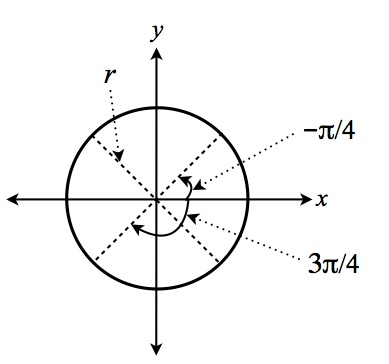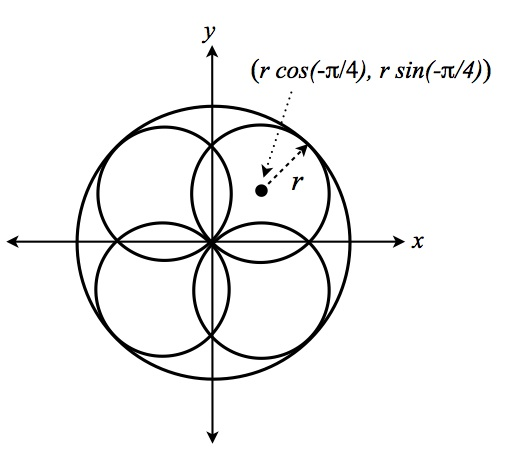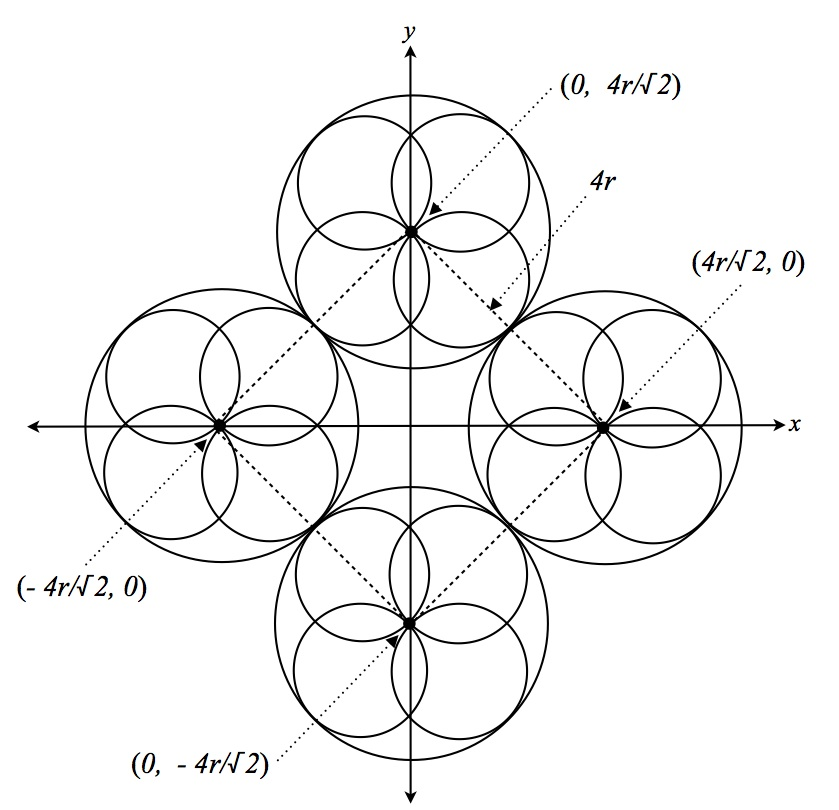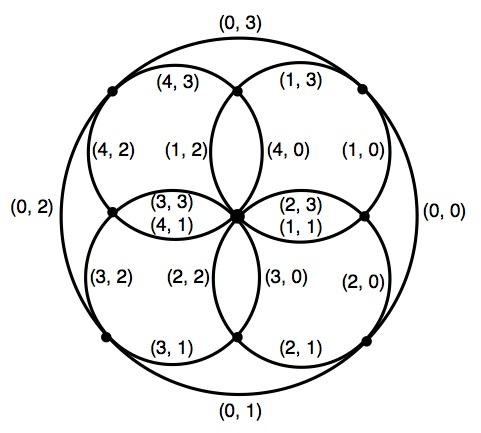## English to Narayan Translator

### Discussion

Figure 2 below shows the underlying geometry of Narayan characters. Each circle, including the circumscribing circle, can be divided into four quarter-arcs. Any Narayan ideographic symbol can be constructed from this geometry by drawing out various quarter-arcs on the smaller circles and on the circumscribing circle. Figure 1 shows how a circle is divided into four equal arcs, each arc comprising a quarter of the circle.

Each quarter-arc in figure 1 may be described mathematically as

Arc(n) = { f(r, n, τ) },  where

f(r, n, τ) = ( fx(r, n, τ), fy(r, n, τ) ),   such that

fx(r, n, τ) = r cos((2(n + τ) - 1)π/4),
fy(r, n, τ) = r sin((2(n + τ) - 1)π/4),
τ ∈ [0, 1],
n ∈ {0, 1, 2, 3}.Figure 1. A circle divided up into quarter-arcs.

A Narayan character may be described mathematically as

Arc(m, n) = { ( fx(r, n, τ) + xm, fy(r, n, τ) + ym ) },   where

xm = r cos((2m - 1)π/4),
ym = r sin((2m - 1)π/4),
τ ∈ [0, 1],
m, n ∈ {0, 1, 2, 3}.
which, when combined with the equation for the circumscribing circle,
Arc(k) = { f(2r, k, τ) },  where

τ ∈ [0, 1],
k ∈ {0, 1, 2, 3}.
completely describes the character.Figure 2. Underlying geometry of a Narayan character.

A Narayan, four word phrase may be constructed by translating each character to its respective cardinal position on a circle of radius 4r/√2. That is, the center of each Narayan character should be located as shown in figure 3. The first character located at the 12:00 position, the second at 3:00, the third at 6:00, and the forth at 9:00.Figure 3. Underlying geometry of a Narayan phrase.

### Code Description

The individual quarter-arc segments that make up a Narayan character are stored in a Javascript object that itself contains a set of objects. The property of each object is a Narayan word; the value is a array of integers. The array is organized into pairs of integers as follows. The first integer identifies a specific circle in the underlying geometry of the character

0, the circumscribing circle,
1, the minor circle in the 1:30 position,
2, the minor circle in the 4:30 position,
3, the minor circle in the 7:30 position,
4, the minor circle in the 10:30 position.

The second integer selects a specific quarter-arc on the circle specified by the first integer

0, the quarter-arc centered in the 3:00 position,
1, the quarter-arc centered in the 6:00 position,
2, the quarter-arc centered in the 9:00 position,
3, the quarter-arc centered in the 12:00 position.
Figure 4 shows how pairs of integers are used to specify the quarter-arcs that make up a Narayan character.Figure 4. Quarter arcs specified by pairs of integers.

For example, referring to figure 4, in the snippet below the first pair for "Balance" [0,1] identifies the quarter-arc segment in the 6:00 position on the circumscribing circle. The first pair for "Believe" [4,3] identifies the quarter-arc segment in the 12:00 position on the minor circle in the 10:30 position.

```var PATTERNS = {
"": [ ],
"Balance": [ 0,1, 0,3, 1,0, 1,1, 3,2, 3,3 ],
"Believe": [ 4,3, 4,2, 4,1, 1,1, 1,0, 0,0, 0,1 ],
"Change": [ 0,3, 0,2, 3,0, 3,1, 3,2, 3,3, 1,2, 1,1, 4,0, 2,3 ],
"Chaos": [ 4,2, 0,3, 1,0, 1,2, 2,3, 2,0, 0,1, 3,1 ],
"Civilization": [ 0,3, 4,2, 4,3, 4,0, 1,2, 1,3, 1,0, 2,2, 2,1,
3,0, 3,1 ],
"Constraint": [ 4,3, 4,2, 4,1, 1,2, 0,1, 0,0, 1,0, 2,0 ],
.
.
.
};
```

The code snippet below shows the Javascript function that draws a Narayan phrase on the HTML canvas. Note that xPos and yPos are the x, y coordinates of the center of each word in the phrase. These coordinates correspond to (0, 4r/√2), (4r/√2, 0),
(0, -4r/√2), etc, as shown above in figure 3.

```function drawSymbol(sWord, xPos, yPos) {
/*
* Description:
*   Draws a Narayan character, centered at the location
*   specified by the supplied coordinates.
* Parameters:
*   sWord - the Narayan character to draw
*   xPos, yPos - the coordinates of where to draw the
*                character
*/
var i;          // iterating variable
var mCenter;    // coordinates for center of minor circle
var piov4;      // a constant equivalent to 45 degrees
var thetaBegin; // start angle of specified quarter-arc
var thetaEnd;   // end angle of specified quarter-arc
var thetaOrg;   // angle of origin of minor circle
var pattern;    // the array specifying the quarter-arcs

pattern = PATTERNS[sWord];
piov4 = Math.PI / 4;

for(i = 0; i < pattern.length; i++) {
/*
* Iterate through pairs of integers in the pattern array
* that describes the Narayan character specified by sWord.
* For each pair define the beginning and end points of
* quarter-arc specified by the second integer in the pair.
*/
thetaBegin = (2 * pattern[i + 1] - 1) * piov4;
thetaEnd = thetaBegin + 2 * piov4;

if(pattern[i] == 0) {
/*
* If the first integer in the pair is 0, then draw on
* circumscribing circle the quarter-arc specified by the
* second integer in the pair.
*/
ctx.beginPath();
ctx.arc(xPos, yPos, 2 * unitRadius, thetaBegin, thetaEnd);
ctx.stroke();
ctx.closePath();
} else {
/*
* If the first integer in the pair specifies a minor circle,
* then get the rectangular coordinates of the center of the
* minor circle specified by the first integer.
*/
thetaOrg = (2 * pattern[i] - 3) * piov4;
/*
* Draw the quarter-arc specified by the second integer
* in the pair.
*/
ctx.beginPath();
ctx.arc(mCenter.x + xPos, mCenter.y + yPos,